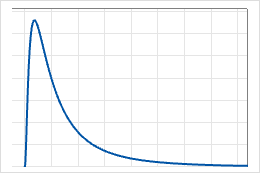# Lognormal distribution

Use the lognormal distribution if the logarithm of the random variable is normally distributed. Use when random variables are greater than 0. For example, the lognormal distribution is used for reliability analysis and in financial applications, such as modeling stock behavior.

The lognormal distribution is a continuous distribution that is defined by its location and scale parameters. The 3-parameter lognormal distribution is defined by its location, scale, and threshold parameters.

The shape of the lognormal distribution is similar to that of the loglogistic and Weibull distributions. For example, the following graph illustrates the lognormal distribution for scale=1.0, location=0.0, and threshold=0.0.By using this site you agree to the use of cookies for analytics and personalized content.  Read our policy